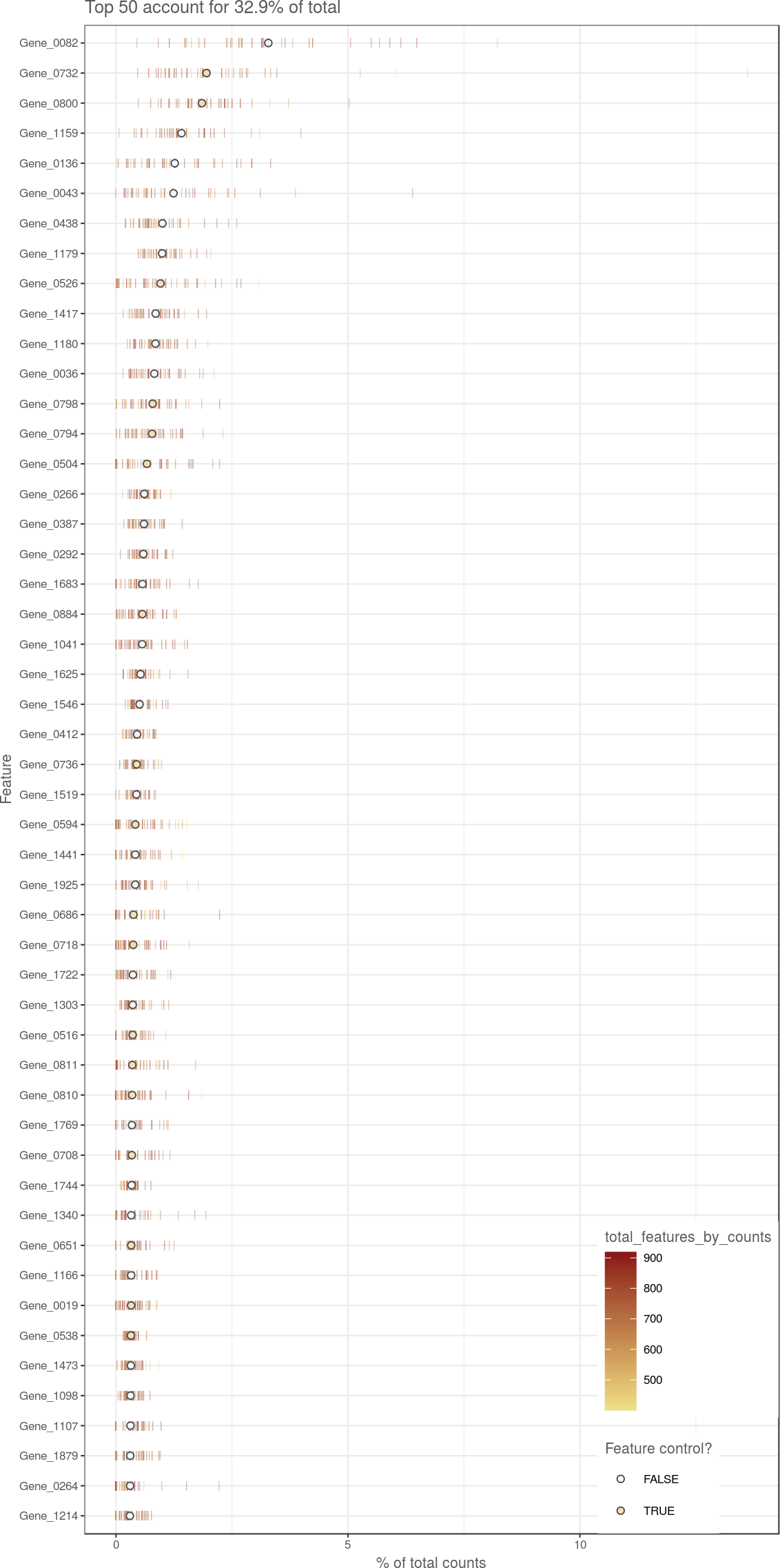# 1 Overview

The `scater` package puts a focus on aiding with quality control (QC) and pre-processing of single-cell RNA-seq data before further downstream analysis. We see QC as consisting of three distinct steps:

1. QC and filtering of cells
2. QC and filtering of features (genes)
3. QC of experimental variables

Following QC, we can proceed with data normalisation before downstream analysis and modelling. We will demonstrate on some example data generated below:

``````library(scater)
data("sc_example_counts")
data("sc_example_cell_info")
example_sce <- SingleCellExperiment(
assays = list(counts = sc_example_counts),
colData = sc_example_cell_info
)
example_sce``````
``````## class: SingleCellExperiment
## dim: 2000 40
## assays(1): counts
## rownames(2000): Gene_0001 Gene_0002 ... Gene_1999 Gene_2000
## rowData names(0):
## colnames(40): Cell_001 Cell_002 ... Cell_039 Cell_040
## colData names(4): Cell Mutation_Status Cell_Cycle Treatment
## reducedDimNames(0):
## spikeNames(0):``````

# 2 Calculating QC metrics

## 2.1 Introducing the `calculateQCMetrics` function

The `calculateQCMetrics` function computes a number of quality control metrics for each cell and feature, stored in the `colData` and `rowData` respectively. By default, the QC metrics are computed from the count data, but this can be changed through the `exprs_values` argument.

``````example_sce <- calculateQCMetrics(example_sce)
colnames(colData(example_sce))``````
``````##   "Cell"                           "Mutation_Status"
##   "Cell_Cycle"                     "Treatment"
##   "is_cell_control"                "total_features_by_counts"
##   "log10_total_features_by_counts" "total_counts"
##   "log10_total_counts"             "pct_counts_in_top_50_features"
##  "pct_counts_in_top_100_features" "pct_counts_in_top_200_features"
##  "pct_counts_in_top_500_features"``````
``colnames(rowData(example_sce))``
``````##  "is_feature_control"    "mean_counts"           "log10_mean_counts"
##  "n_cells_by_counts"     "pct_dropout_by_counts" "total_counts"
##  "log10_total_counts"``````

Control sets can be defined for features (e.g., spike-in transcripts, mitochondrial genes) or cells (e.g., empty wells, visually damaged cells). The function will subsequently compute metrics for each control set, e.g., the proportion of counts assigned to spike-in transcripts.

``````example_sce <- calculateQCMetrics(example_sce,
feature_controls = list(ERCC = 1:20, mito = 500:1000),
cell_controls = list(empty = 1:5, damaged = 31:40))

all_col_qc <- colnames(colData(example_sce))
all_col_qc <- all_col_qc[grep("ERCC", all_col_qc)]``````

Metrics are also computed for the union of all control sets, and for the set of all features/cells that are not marked as controls. These sets are labelled as `"feature_control"` and `"endogenous"` for features, and `"cell_control"` and `"non_control"` for cells. Users should avoid using these names for their own sets.

## 2.2 Cell-level QC metrics

We refer users to the `?calculateQCMetrics` for a full list of the computed cell-level metrics. However, we will describe some of the more popular ones here:

• `total_counts`: total number of counts for the cell (i.e., the library size).
• `total_features_by_counts`: the number of features for the cell that have counts above the detection limit (default of zero).
• `pct_counts_X`: percentage of all counts that come from the feature control set named X.

If `exprs_values` is set to something other than `"counts"`, the names of the metrics will be changed by swapping `"counts"` for whatever named assay was used.

## 2.3 Feature-level QC metrics

Feature-level metrics include:

• `mean_counts`: the mean count of the gene/feature.
• `pct_dropout_by_counts`: the percentage of cells with counts of zero for each gene.
• `pct_counts_Y`: percentage of all counts that come from the cell control set named Y.

Again, if a different `exprs_values` was used, the names of the metrics will change correspondingly.

# 3 Producing diagnostic plots for QC

## 3.1 Examining the most expressed features

We look at a plot that shows the top 50 (by default) most-expressed features. Each row in the plot below corresponds to a gene, and each bar corresponds to the expression of a gene in a single cell. The circle indicates the median expression of each gene, with which genes are sorted. By default, “expression” is defined using the feature counts (if available), but other expression values can be used instead by changing `exprs_values`.

``plotHighestExprs(example_sce, exprs_values = "counts")``We expect to see the “usual suspects”, i.e., mitochondrial genes, actin, ribosomal protein, MALAT1. A few spike-in transcripts may also be present here, though if all of the spike-ins are in the top 50, it suggests that too much spike-in RNA was added. A large number of pseudo-genes or predicted genes may indicate problems with alignment.

## 3.2 Frequency of expression as a function of the mean

Another useful plot is that of the frequency of expression (i.e., number of cells with non-zero expression) against the mean. These two metrics should be positively correlated with each other for most genes.

``plotExprsFreqVsMean(example_sce)``